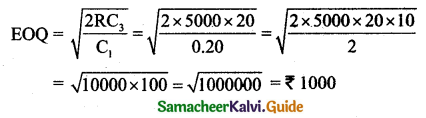Tamilnadu State Board New Syllabus Samcheer Kalvi 11th Business Maths Guide Pdf Chapter 6 Applications of Differentiation Ex 6.6 Text Book Back Questions and Answers, Notes.

## Tamilnadu Samacheer Kalvi 11th Business Maths Solutions Chapter 6 Applications of Differentiation Ex 6.6

### Samacheer Kalvi 11th Business Maths Applications of Differentiation Ex 6.6 Text Book Back Questions and Answers

Question 1.
Average fixed cost of the cost function C(x) = 2x3 + 5x2 – 14x + 21 is:
(a) $$\frac{2}{3}$$
(b) $$\frac{5}{x}$$
(c) $$-\frac{14}{x}$$
(d) $$\frac{21}{x}$$
(d) $$\frac{21}{x}$$
Hint:
Average fixed cost $$\frac{k}{x}=\frac{21}{x}$$Question 2.
Marginal revenue of the demand function p = 20 – 3x is:
(a) 20 – 6x
(b) 20 – 3x
(c) 20 + 6x
(d) 20 + 3x
(a) 20 – 6x
Hint:
p = 20 – 3x
Revenue, R = px = (20 – 3x)x = 20x – 3x2
Marginal revenue = $$\frac{d \mathrm{R}}{d x}$$ = 20 – 6x

Question 3.
If demand and the cost function of a firm are p = 2 – x and C = -2x2 + 2x + 7 then its profit function is:
(a) x2 + 7
(b) x2 – 7
(c) -x2 + 7
(d) -x2 – 7
(b) x2 – 7
Hint:
Profit = Revenue – Cost
= (2 – x)x – (-2x2 + 2x + 7)
= 2x – x2 + 2x2 – 2x – 7
= x2 – 7Question 4.
If the demand function is said to be inelastic, then:
(a) |ηd| > 1
(b) |ηd| = 1
(c) |ηd| < 1
(d) |ηd| = 0
(a) |ηd| > 1

Question 5.
The elasticity of demand for the demand function x = $$\frac{1}{p}$$ is:
(a) 0
(b) 1
(c) $$-\frac{1}{p}$$
(d) ∞
(b) 1
Hint: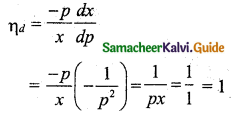Question 6.
Relationship among MR, AR and ηd is:
(a) $$\eta_{d}=\frac{\mathrm{AR}}{\mathrm{AR}-\mathrm{MR}}$$
(b) ηd = AR – MR
(c) MR = AR = ηd
(d) AR = $$\frac{\mathrm{MR}}{\eta_{d}}$$
(a) $$\eta_{d}=\frac{\mathrm{AR}}{\mathrm{AR}-\mathrm{MR}}$$

Question 7.
For the cost function C = $$\frac{1}{25} e^{5x}$$, the marginal cost is:
(a) $$\frac{1}{25}$$
(b) $$\frac{1}{5} e^{5 x}$$
(c) $$\frac{1}{125} e^{5 x}$$
(d) 25e5x
(b) $$\frac{1}{5} e^{5 x}$$
Hint:
C = $$\frac{1}{25} e^{5x}$$
Marginal cost $$\frac{d c}{d x}=\frac{1}{25}\left(5 e^{5 x}\right)=\frac{1}{5} e^{5 x}$$Question 8.
Instantaneous rate of change of y = 2x2 + 5x with respect to x at x = 2 is:
(a) 4
(b) 5
(c) 13
(d) 9
(c) 13
Hint:
y = 2x2 + 5x
$$\frac{d y}{d x}$$ = 4x + 5
$$\left(\frac{d y}{d x}\right)_{x=2}$$ = 4 × 2 + 5 = 13

Question 9.
If the average revenue of a certain firm is ₹ 50 and its elasticity of demand is 2, then their marginal revenue is:
(a) ₹ 50
(b) ₹ 25
(c) ₹ 100
(d) ₹ 75
(b) ₹ 25
Hint: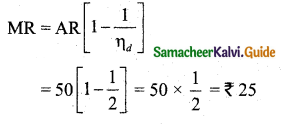Question 10.
Profit P(x) is maximum when:
(a) MR = MC
(b) MR = 0
(c) MC = AC
(d) TR = AC
(a) MR = MC

Question 11.
The maximum value of f(x) = sin x is:
(a) 1
(b) $$\frac{\sqrt{3}}{2}$$
(c) $$\frac{1}{\sqrt{2}}$$
(d) $$-\frac{1}{\sqrt{2}}$$
(a) 1
Hint:
When x = $$\frac{\pi}{2}$$, sin x is maximum.
Maximum value is sin$$\frac{\pi}{2}$$ = 1Question 12.
If f(x, y) is a homogeneous function of degree n, then $$x \frac{\partial f}{\partial x}+y \frac{\partial f}{\partial y}$$ is equal to:
(a) (n – 1)f
(b) n(n – 1)f
(c) nf
(d) f
(c) nf

Question 13.
If u = 4x2 + 4xy + y2 + 4x + 32y + 16, then $$\frac{\partial^{2} u}{\partial y \partial x}$$ is equal to:
(a) 8x + 4y + 4
(b) 4
(c) 2y + 32
(d) 0
(b) 4
Hint:
u = 4x2 + 4xy + y2 + 4x + 32y + 16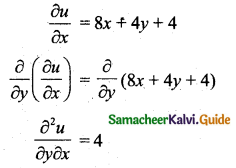Question 14.
If u = x3 + 3xy2 + y3 then $$\frac{\partial^{2} u}{\partial y \partial x}$$ is:
(a) 3
(b) 6y
(c) 6x
(d) 2
(b) 6y
Hint:
u = x3 + 3xy2 + y3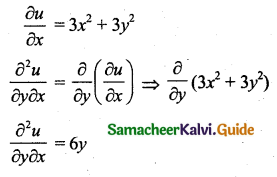Question 15.
If u = $$e^{x^{2}}$$ then $$\frac{\partial u}{\partial x}$$ is equal to:
(a) $$2 x e^{x^{2}}$$
(b) $$e^{x^{2}}$$
(c) $$2 e^{x^{2}}$$
(d) 0
(a) $$2 x e^{x^{2}}$$
Hint:
u = $$e^{x^{2}}$$
$$\frac{\partial u}{\partial x}=2 x e^{x^{2}}$$Question 16.
Average cost is minimum when:
(a) Marginal cost = marginal revenue
(b) Average cost = marginal cost
(c) Average cost = Marginal revenue
(d) Average Revenue = Marginal cost
(b) Average cost = marginal cost

Question 17.
A company begins to earn profit at:
(a) Maximum point
(b) Breakeven point
(c) Stationary point
(d) Even point
(b) Breakeven pointQuestion 18.
The demand function is always:
(a) Increasing function
(b) Decreasing function
(c) Non-decreasing function
(d) Undefined function
(b) Decreasing function

Question 19.
If q = 1000 + 8p1 – p2 then, $$\frac{\partial q}{\partial p_{1}}$$ is:
(a) -1
(b) 8
(c) 1000
(d) 1000 – p2
(b) 8
Hint:
q = 1000 + 8p1 – p2
$$\frac{8 q}{\partial p_{1}}$$ = 8Question 20.
If R = 5000 units/year, C1 = 20 paise, C3 = ₹ 20 then EOQ is:
(a) 5000
(b) 100
(c) 1000
(d) 200